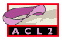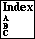## O-P

a recognizer for the ordinals up to epsilon-0
```Major Section:  PROGRAMMING
```

Using the nonnegative integers and lists we can represent the ordinals up to `epsilon-0`. The ordinal representation used in ACL2 has changed as of Version_2.8 from that of Nqthm-1992, courtesy of Pete Manoilios and Daron Vroon; additional discussion may be found in ``Ordinal Arithmetic in ACL2'', proceedings of ACL2 Workshop 2003, `http://www.cs.utexas.edu/users/moore/acl2/workshop-2003/`. Previously, ACL2's notion of ordinal was very similar to the development given in ``New Version of the Consistency Proof for Elementary Number Theory'' in The Collected Papers of Gerhard Gentzen, ed. M.E. Szabo, North-Holland Publishing Company, Amsterdam, 1969, pp 132-213.

The following essay is intended to provide intuition about ordinals. The truth, of course, lies simply in the ACL2 definitions of `o-p` and `o<`.

Very intuitively, think of each non-zero natural number as by being denoted by a series of the appropriate number of strokes, i.e.,

```0             0
1             |
2             ||
3             |||
4             ||||
...           ...
```
Then ```omega`,'' here written as `w`, is the ordinal that might be written as
```w             |||||...,
```
i.e., an infinite number of strokes. Addition here is just concatenation. Observe that adding one to the front of `w` in the picture above produces `w` again, which gives rise to a standard definition of `w`: `w` is the least ordinal such that adding another stroke at the beginning does not change the ordinal.

We denote by `w+w` or `w*2` the ```doubly infinite`'' sequence that we might write as follows.

```w*2           |||||... |||||...
```
One way to think of `w*2` is that it is obtained by replacing each stroke in `2` `(||)` by `w`. Thus, one can imagine `w*3`, `w*4`, etc., which leads ultimately to the idea of ```w*w`,'' the ordinal obtained by replacing each stroke in `w` by `w`. This is also written as ```omega` squared'' or `w^2`, or:
``` 2
w             |||||... |||||... |||||... |||||... |||||... ...
```
We can analogously construct `w^3` by replacing each stroke in `w` by `w^2` (which, it turns out, is the same as replacing each stroke in `w^2` by `w`). That is, we can construct `w^3` as `w` copies of `w^2`,
``` 3              2       2       2       2
w              w  ...  w  ...  w  ...  w ... ...
```
Then we can construct `w^4` as `w` copies of `w^3`, `w^5` as `w` copies of `w^4`, etc., ultimately suggesting `w^w`. We can then stack `omega`s, i.e., `(w^w)^w` etc. Consider the ``limit'' of all of those stacks, which we might display as follows.
```       .
.
.
w
w
w
w
w
```
That is epsilon-0.

Below we begin listing some ordinals up to `epsilon-0`; the reader can fill in the gaps at his or her leisure. We show in the left column the conventional notation, using `w` as ```omega`,'' and in the right column the ACL2 object representing the corresponding ordinal.

```  ordinal            ACL2 representation

0                  0
1                  1
2                  2
3                  3
...                ...
w                 '((1 . 1) . 0)
w+1               '((1 . 1) . 1)
w+2               '((1 . 1) . 2)
...                ...
w*2               '((1 . 2) . 0)
(w*2)+1           '((1 . 2) . 1)
...                ...
w*3               '((1 . 3) . 0)
(w*3)+1           '((1 . 3) . 1)
...                ...

2
w                 '((2 . 1) . 0)
...                ...

2
w +w*4+3          '((2 . 1) (1 . 4) . 3)
...                ...

3
w                 '((3 . 1) . 0)
...                ...

w
w                 '((((1 . 1) . 0) . 1) . 0)
...                ...

w  99
w +w  +w4+3       '((((1 . 1) . 0) . 1) (99 . 1) (1 . 4) . 3)
...                ...

2
w
w                 '((((2 . 1) . 0) . 1) . 0)

...                ...

w
w
w                 '((((((1 . 1) . 0) . 1) . 0) . 1) . 0)
...               ...
```
Observe that the sequence of `o-p`s starts with the natural numbers (which are recognized by `natp`). This is convenient because it means that if a term, such as a measure expression for justifying a recursive function (see o<) must produce an `o-p`, it suffices for it to produce a natural number.

The ordinals listed above are listed in ascending order. This is the ordering tested by `o<`.

The ```epsilon-0` ordinals'' of ACL2 are recognized by the recursively defined function `o-p`. The base case of the recursion tells us that natural numbers are `epsilon-0` ordinals. Otherwise, an `epsilon-0` ordinal is a list of `cons` pairs whose final `cdr` is a natural number, `((a1 . x1) (a2 . x2) ... (an . xn) . p)`. This corresponds to the ordinal `(w^a1)x1 + (w^a2)x2 + ... + (w^an)xn + p`. Each `ai` is an ordinal in the ACL2 representation that is not equal to 0. The sequence of the `ai`'s is strictly decreasing (as defined by `o<`). Each `xi` is a positive integer (as recognized by `posp`).

Note that infinite ordinals should generally be created using the ordinal constructor, `make-ord`, rather than `cons`. The functions `o-first-expt`, `o-first-coeff`, and `o-rst` are ordinals destructors. Finally, the function `o-finp` and the macro `o-infp` tell whether an ordinal is finite or infinite, respectively.

The function `o<` compares two `epsilon-0` ordinals, `x` and `y`. If both are integers, `(o< x y)` is just `x<y`. If one is an integer and the other is a `cons`, the integer is the smaller. Otherwise, `o<` recursively compares the `o-first-expt`s of the ordinals to determine which is smaller. If they are the same, the `o-first-coeff`s of the ordinals are compared. If they are equal, the `o-rst`s of the ordinals are recursively compared.

Fundamental to ACL2 is the fact that `o<` is well-founded on `epsilon-0` ordinals. That is, there is no ``infinitely descending chain'' of such ordinals. See proof-of-well-foundedness.